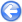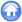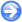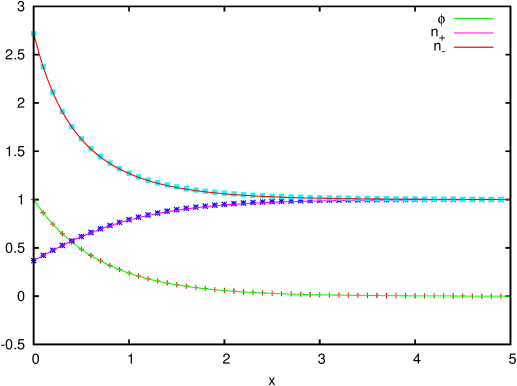### 14.5PASS: Gouy-Chapman Debye layer

Author
J.M. López-Herrera
Command
sh debye.sh
Version
111123
Required files
debye.sh points analytical
Running time
12 seconds

The Debye layer is the ionic concentration and potential distribution equilibrium structure that appears on the surface of a charged electrode in contact with solvents in which are dissolved ionic species. Gouy and Chapman proposed a model of a diffuse Debye layer taking into account that the concentrations of these ionic species are governed by the combined effect of its thermal diffusion and its electrostatic attraction or repulsion.

In the case of a plane electrode within a fully dissolved binary system (ion and counterions of valence z, |z|=1) the model reduces to the following dimensionless equations:

 n+ = e−φ    and    n−=eφ
 d2 φ/d x2 = −(n+−n−)=2 sinh(φ)

with the boundary conditions: φ(0)=φo and φ(x → ∞)=0. In the above equations the concentrations of anions and cations, n+ and n respectively, have been made dimensionless with the bulk concentration, no, the potential φ with (Kb T/e) and lengths with the Debye’s length λD = [no e2/(Kb T ε)]1/2 where Kb is Boltzmann’s constant, T the temperature, e the charge of the electron and ε the permittivity of the fluid.

 Figure 211: Profiles of electric potential, concentration of anions and cations in the Debye layer. The lines are the analytical solution and the symbols the numerical solution.